9-8.

Missing problem for Book CC3 => Chapter Ch9 => Lesson 9.1.1 => Problem 9-8

Created from orphaned homework help problem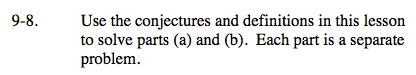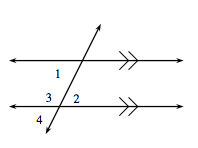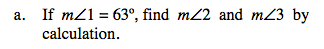Angle 1 and angle 2 are alternate interior angles.

When the lines are parallel, alternate interior angles have equal measures.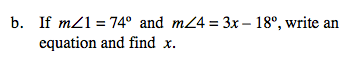Disregard your calculations for angle measure from part (a).

3x − 18 = 74

3x = 92

x = 30.6°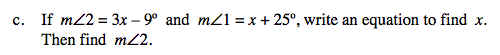Since the lines are parallel, angles 1 and 2 have equal measure.

x = 17°, m∠2 = 42°